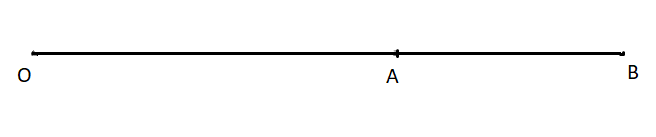Daily Practice Problems
Class 12 Physics
Electric Charges and FieldsQuestion 1:

A point charge Q is placed at point O as shown in figure. Is the potential difference VA- VB positive, negative or zero; If Q is (i) positive (ii) negative

q+1. Positive, negative
2. Negative, positive
3. Positive, positive
4. Negative, negative

Question 2:

8 dipoles of charges of magnitude ± e is placed inside a cube. What will be the total flux coming out of the cube?

1. 0
2. Α
3. e
4. -e

Question 3:

When air is replaced by a dielectric medium of dielectric constant K, the maximum force of attraction between two charges separated by a distance?

1. Decreases k times
2. Remains unchanged
3. Increase k times
4. Decreases k2 times

Question 4:

An electron is moving with a constant velocity v along X- axis enters a uniform electric field applied along Y-axis. Then the electron moves

1. With uniform acceleration along Y-axis
2. Without uniform acceleration along Y-axis
3. In a trajectory represented as y= ax2
4. In a trajectory represented as y=ax

Question 5:

An ideal electric dipole has

1. Equal and similar charged separated by small distance.
2. Unequal and opposite charges separated by large distance.
3. Equal and opposite charges separated by negligibly small distance
4. Equal and opposite charges separated by negligibly small distance.

Question 6:

The electric flux through the surface1. In fig. (iv) is the largest
2. In fig. (iii) is the least
3. In fig. (ii) is same as fig.(iii) but is smaller than fig.(iv)
4. Is the same for all the figures.

Question 7:

Find the amount of work in rotating a dipole of dipole moment 3×10-3 cm from the position of stable equilibrium to the position od unstable equilibrium, in a uniform electric field of intensity 104 NC-1.

Question 8:

Two metal plates having a potential difference of 800 V are 0.02 m apart horizontally. A particle of mass 1.96 × 10-15 kg is suspended in equilibrium between the plates. If e is elementary charges, then charge on the particle is,

Question 9:

An α particle and a proton are accelerated at same potential difference from rest. Find the ratio of their velocities.

Question 10:

1. An electrostatic field line is a continuous curve. That is, a field line cannot have sudden breaks. Why not?
2. Explain why two field lines never cross each other at any point?

Question 11:

What is meant by the statement that the electric field of a point charge has spherical symmetry whereas that of an electric dipole is cylindrically symmetrical?

Question 12:

The electrostatic force of repulsion between two positively charged ions carrying equal charge is 3.7 × 10-9 N, when they are separated by a distance of 5Å . How many electrons are missing from each ion?

Question 13:

State and prove Gauss Theorem.

Question 14:

A particle of mass m carrying charge +q1 is revolving around a fixed -q2 in a circular path of radius r. Calculate the period of revolution.

Directions: These questions consist of two statements, each printed as Assertion and Reason. While answering these questions, you are required to choose any one of the following four responses.

(a) Both Assertion and Reason are correct and the Reason is a correct explanation of the Assertion.

(b) Both Assertion and Reason are correct but Reason is not a correct explanation of the Assertion.

(c) Assertion is correct, Reason is incorrect

(d) Assertion is incorrect and Reason is correct.

(e) Both assertion and reason are incorrect.

Question 15:

Assertion (A): A parallel plates charged capacitor is disconnected from a battery. Now if its plates are separated farther, the potential fall.

Reason (R): Energy stored in a capacity is equal to the work done in charging it.

Question 16:

Assertion: A uniformly charged ring behaves like a large charge if the point of observation is far away from the ring.

Reason: Electrostatic potential energy is maximum when the dipole is aligned parallel to the direction of Electric field.

Question 17:

Assertion: No work is done in moving a test charge between two points on an equilibrium surface.

Reason: Electric field acts normally to the equipotential surface.

Question 18:

Assertion: A lightning conductor at the top of high buildings are sharp pointed ends.

Reason: the surface charge density at sharp points is very high resulting in setting up of electric wind.

Question 19:

Assertion: An ebonite rod held in hand can be charged by rubbing with wool but copper rod cannot be charged like this.

Reason: Ebonite is good conductor of electricity, the charges so produced stay on ebonite rod.

Question 20:

Assertion: The tyres of aircrafts are slightly conducting.

Reason: If a conductor is connected to the ground, the extra charge induced on conductor will flow to the ground.

**********